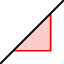# Parallel and Perpendicular Lines in the Coordinate Plane

## DIRECTIONS:

In the applet window below, 1) Construct a line. 2) Construct a line parallel to this given line. 3) Use the SLOPE toolto measure and display the slopes of both lines.

## 4)

Select the MOVE tool again. Move any of the first 2 points you plotted (in step 1) around. What do you notice?

5) Construct a line that is perpendicular to the first line you drew that passes through any other point It doesn't matter where you choose to position this line. 6) Use the SLOPE toolto measure and display the slope of this perpendicular line.

## Continued from above...

7) The label for the slope of the first line should be . The label for the slope of the perpendicular line should be . Go to the "Steps" window. In the next input line, type (w/o the " "): "FractionText(m)". In the next input line, type (w/o the " "): "FractionText". If you so choose, you can choose to show these text boxes in the graphics window.

## 8)

Move any one of the first 2 points you plotted (in step 1) around. What do you notice about the slope of this line and the line perpendicular to it? Explain.